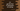# C# program to get the first N characters of a string## C# program to get the first N characters of a string:

In this post, we will learn how to get or print the first N characters or the substring containing the first N characters of a string in C#.

For example, if the string is Hello and if we want the substring holding the first 2 characters of this string, it will print He.

Let’s take a look at the way to do that with an example.

## String.Substring method:

String class provides one method called Substring to retrieve a substring from a string. This method can be used to get one substring holding the first N characters of a string.

This method is defined as below:

``public string Substring (int startIndex, int length);``

It takes two arguments. The first one, startIndex is the starting character position. length is the length of the substring. In our case length is N.

It will return one substring of size length. This substring starts at index startIndex. Or Empty if the startIndex is equal to the size of the string and length is 0.

### Exception:

String.Substring method throws an exception if any of these two values is invalid. For example, they can’t be less than zero and startIndex and length should always give a valid substring.

### C# program to get the substring holding the first N characters:

In our case, the startIndex is 0 since we need only the start substring. Below is the complete program:

``````using System;

public class Program
{
public static string getSubStr(String str, int N){
if (string.IsNullOrEmpty(str)){
return str;
}

return str.Substring(0, Math.Min(str.Length, N));
}

public static void Main()
{
String givenString = "Hello";

Console.WriteLine(getSubStr(givenString, 3));
}
}``````

Here,

• We are using getSubStr method to get the final substring.
• It takes two parameters. The first one is the string to check and the second one is the value of N, i.e. the number of characters for the substring.
• It first checks if the provided string is null or empty. If yes, it returns the same string. Else it uses Substring method to get the substring.

• Note that we are using Math.Min to find the minimum value between the length and N. Otherwise, it will crash the code.

If you run the above program, it will give the bellow output:

``Hel``